# Twenty five coloured beads are to be arranged - CAT DILR questions – Logical Reasoning

## CAT 2020 - Slot 2 - Question 1 - Twenty five coloured beads are to be arranged

Q1. The total number of possible congurations using beads of only two colours is: (TITA)

1. 2.

Since only 2 beads can be used, Red is automatically out as it requires at least 1 G and 1 B to be between 2 Rs

Given the condition that there can be no beads of the same colour adjacent to each other, we can have 1 of 2 arrangements: Either B in the corner or G in the corner

So only 2 arrangements are possible here (This can be concluded without actually drawing the grid, so a must attempt question)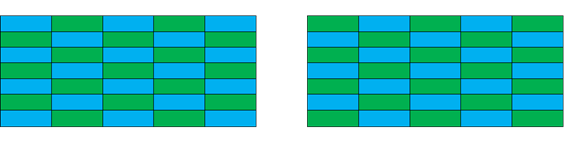Q2. What is the maximum possible number of Red beads that can appear in any conguration? (TITA)

2. 9.

The idea here is to maximize the number of Reds in each row, this question will require us to draw the grid

Step 1: Try to draw as many Rs in a row as possible, since we know that 1R needs to have at least 1B and 1G between them, to maximize, we will need to have (ideally) exactly 1B and 1G

Move along the diagonal as you are trying to fill the Red beads since that way, we can also ensure that 2Rs do not come in contact in adjacent cells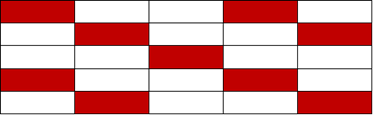Step 2: Let’s see if this is feasible with the given constraints. We will now need to fill in the G and B to see if it is feasible.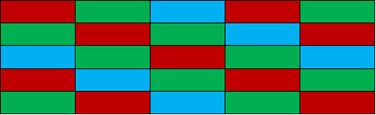It is indeed feasible, by now you would have already noticed a pattern that moving along the diagonal (especially because of the non-repeating colours condition) is absolutely crucial to understanding the pattern in the grid. Here as well, you can see that the Bs and Gs are along the diagonal.

Q3. What is the minimum number of Blue beads in any conguration? (TITA)

3. 6.

The previous solution if done properly should have already answered this question. But if the previous one was not attempted then here is the way to solve this:

We need to include a minimum number of Bs in the grid, meaning, ideally we should include only 1 B per row. Let’s attempt to do that.

Before we begin, what seems to be clear from the grid so far is that, if we are trying to maximize any colour, it is possible only if we move through the main diagonal. Clearly since that is not what we are trying to achieve for Bs, we should make sure that in the initial arrangement, B is not present in the middle cell / along the main diagonal

But while filling only 1 colour, we always move through the diagonal of the starting point. An initial arrangement might look like this: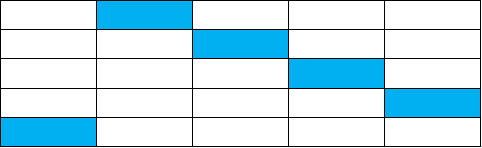Now let’s try to fill in colours and see what is feasible. While doing so, we need to keep in mind that since we are minimizing B, it automatically means we are trying to maximize R and G. Which means that along the main diagonal should be beads with either R or G: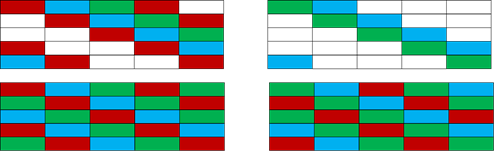It might be tempting to stop here and answer 7. But we also need to try the other initial arrangement where we move B through the other remaining diagonal: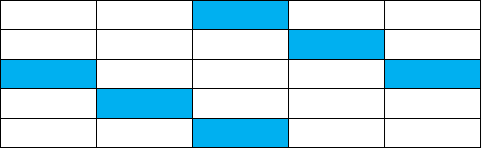Let’s try the same method of filling the main diagonal with either R or G. It is not feasible to use G in the main diagonal (can find out quickly by filling in the grid)

So the only feasible solution is: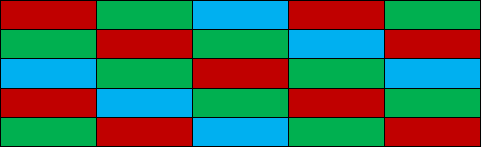Q4. Two Red beads have been placed in ‘second row, third column’ and ‘third row, second column’. How many more Red beads can be placed so as to maximise the number of Red beads used in the configuration? (TITA)

4. 6

The initial configuration is: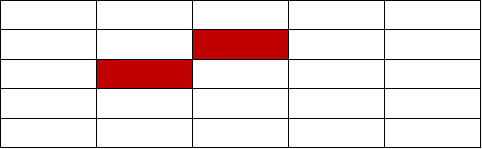To maximize the Rs, we need to move along the diagonal and also add R wherever we can see a space of 2 cells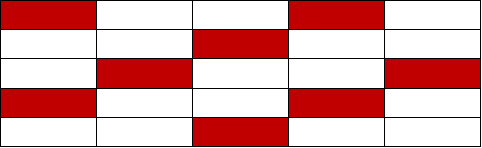We can simply stop here and directly answer 6. But just to confirm: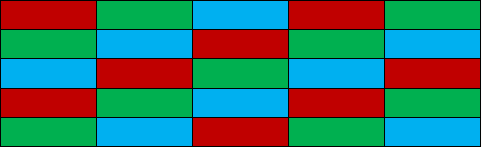### Past Year Question Paper & SolutionsCounselling Session
By IIM Mentor

#### Free Material Area